# 1 Performing variable selection

• Forward selection, backward elimination, and branch and bound selection can be done using VariableSelection().
• VariableSelection() can accept either a BranchGLM object or a formula along with the data and the desired family and link to perform the variable selection.
• Available metrics are AIC, BIC and HQIC, which are used to compare models and to select the best models.
• VariableSelection() returns some information about the search, more detailed information about the best models can be seen by using the summary() function.
• Note that VariableSelection() will properly handle interaction terms and categorical variables.
• keep can also be specified if any set of variables are desired to be kept in every model.

## 1.1 Metrics

• The 3 different metrics available for comparing models are the following
• Akaike information criterion (AIC), which typically results in models that are useful for prediction
• $$AIC = -2logLik + 2 * p$$
• Bayesian information criterion (BIC), which results in models that are more parsimonious than those selected by AIC
• $$BIC = -2logLik + \log{(n)} * p$$
• Hannan-Quinn information criterion (HQIC), which is in the middle of AIC and BIC
• $$HQIC = -2logLik + 2 * \log({\log{(n)})} * p$$

## 1.2 Stepwise methods

• Forward selection and backward elimination are both stepwise variable selection methods.
• They are not guaranteed to find the best model or even a good model, but they are very fast.
• Forward selection is recommended if the number of variables is greater than the number of observations or if many of the larger models don’t converge.
• These methods will only return 1 best model.
• Parallel computation can be used for the methods, but is generally only necessary for large datasets.

### 1.2.1 Forward selection example

# Loading BranchGLM package
library(BranchGLM)

# Fitting gamma regression model
cars <- mtcars

# Fitting gamma regression with inverse link
GammaFit <- BranchGLM(mpg ~ ., data = cars, family = "gamma", link = "inverse")

# Forward selection with mtcars
forwardVS <- VariableSelection(GammaFit, type = "forward")
forwardVS
#> Variable Selection Info:
#> ------------------------
#> Variables were selected using forward selection with AIC
#> The best value of AIC obtained was 142.2
#> Number of models fit: 27
#>
#> Order the variables were added to the model:
#>
#> 1). wt
#> 2). hp

## Getting final model
fit(summary(forwardVS), which = 1)
#> Results from gamma regression with inverse link function
#> Using the formula mpg ~ hp + wt
#>
#>               Estimate         SE      t  p.values
#> (Intercept) -8.923e-03  2.806e-03 -3.180 0.0034910 **
#> hp          -8.887e-05  2.110e-05 -4.212 0.0002245 ***
#> wt          -9.826e-03  1.384e-03 -7.100  8.21e-08 ***
#> ---
#> Signif. codes:  0 '***' 0.001 '**' 0.01 '*' 0.05 '.' 0.1 ' ' 1
#>
#> Dispersion parameter taken to be 0.0104
#> 32 observations used to fit model
#> (0 observations removed due to missingness)
#>
#> Residual Deviance: 0.33 on 29 degrees of freedom
#> AIC: 142.2
#> Algorithm converged in 3 iterations using Fisher's scoring

### 1.2.2 Backward elimination example

# Backward elimination with mtcars
backwardVS <- VariableSelection(GammaFit, type = "backward")
backwardVS
#> Variable Selection Info:
#> ------------------------
#> Variables were selected using backward elimination with AIC
#> The best value of AIC obtained was 141.9
#> Number of models fit: 49
#>
#> Order the variables were removed from the model:
#>
#> 1). vs
#> 2). drat
#> 3). am
#> 4). disp
#> 5). carb
#> 6). cyl

## Getting final model
fit(summary(backwardVS), which = 1)
#> Results from gamma regression with inverse link function
#> Using the formula mpg ~ hp + wt + qsec + gear
#>
#>               Estimate         SE      t  p.values
#> (Intercept) -4.691e-02  1.782e-02 -2.633   0.01384 *
#> hp          -6.284e-05  3.015e-05 -2.084   0.04675 *
#> wt          -9.485e-03  1.819e-03 -5.213 1.719e-05 ***
#> qsec         1.299e-03  7.471e-04  1.739   0.09348 .
#> gear         2.662e-03  1.652e-03  1.612   0.11870
#> ---
#> Signif. codes:  0 '***' 0.001 '**' 0.01 '*' 0.05 '.' 0.1 ' ' 1
#>
#> Dispersion parameter taken to be 0.0091
#> 32 observations used to fit model
#> (0 observations removed due to missingness)
#>
#> Residual Deviance: 0.29 on 27 degrees of freedom
#> AIC: 141.9
#> Algorithm converged in 3 iterations using Fisher's scoring

## 1.3 Branch and bound

• The branch and bound methods can be much slower than the stepwise methods, but they are guaranteed to find the best models.
• The branch and bound methods are typically much faster than an exhaustive search and can also be made even faster if parallel computation is used.

### 1.3.1 Branch and bound example

• If showprogress is true, then progress of the branch and bound algorithm will be reported occasionally.
• Parallel computation can be used with these methods and can lead to very large speedups.
# Branch and bound with mtcars
VS <- VariableSelection(GammaFit, type = "branch and bound", showprogress = FALSE)
VS
#> Variable Selection Info:
#> ------------------------
#> Variables were selected using branch and bound selection with AIC
#> The best value of AIC obtained was 141.9
#> Number of models fit: 56

## Getting final model
fit(summary(VS), which = 1)
#> Results from gamma regression with inverse link function
#> Using the formula mpg ~ hp + wt + qsec + gear
#>
#>               Estimate         SE      t  p.values
#> (Intercept) -4.691e-02  1.782e-02 -2.633   0.01384 *
#> hp          -6.284e-05  3.015e-05 -2.084   0.04675 *
#> wt          -9.485e-03  1.819e-03 -5.213 1.719e-05 ***
#> qsec         1.299e-03  7.471e-04  1.739   0.09348 .
#> gear         2.662e-03  1.652e-03  1.612   0.11870
#> ---
#> Signif. codes:  0 '***' 0.001 '**' 0.01 '*' 0.05 '.' 0.1 ' ' 1
#>
#> Dispersion parameter taken to be 0.0091
#> 32 observations used to fit model
#> (0 observations removed due to missingness)
#>
#> Residual Deviance: 0.29 on 27 degrees of freedom
#> AIC: 141.9
#> Algorithm converged in 3 iterations using Fisher's scoring
• A formula with the data and the necessary BranchGLM fitting information can also be used instead of supplying a BranchGLM object.
# Can also use a formula and data
formulaVS <- VariableSelection(mpg ~ . ,data = cars, family = "gamma",
link = "inverse", type = "branch and bound",
showprogress = FALSE, metric = "AIC")
formulaVS
#> Variable Selection Info:
#> ------------------------
#> Variables were selected using branch and bound selection with AIC
#> The best value of AIC obtained was 141.9
#> Number of models fit: 56

## Getting final model
fit(summary(formulaVS), which = 1)
#> Results from gamma regression with inverse link function
#> Using the formula mpg ~ hp + wt + qsec + gear
#>
#>               Estimate         SE      t  p.values
#> (Intercept) -4.691e-02  1.782e-02 -2.633   0.01384 *
#> hp          -6.284e-05  3.015e-05 -2.084   0.04675 *
#> wt          -9.485e-03  1.819e-03 -5.213 1.719e-05 ***
#> qsec         1.299e-03  7.471e-04  1.739   0.09348 .
#> gear         2.662e-03  1.652e-03  1.612   0.11870
#> ---
#> Signif. codes:  0 '***' 0.001 '**' 0.01 '*' 0.05 '.' 0.1 ' ' 1
#>
#> Dispersion parameter taken to be 0.0091
#> 32 observations used to fit model
#> (0 observations removed due to missingness)
#>
#> Residual Deviance: 0.29 on 27 degrees of freedom
#> AIC: 141.9
#> Algorithm converged in 3 iterations using Fisher's scoring

### 1.3.2 Using bestmodels

• The bestmodels argument can be used to find the top k models according to the metric.
# Finding top 10 models
formulaVS <- VariableSelection(mpg ~ . ,data = cars, family = "gamma",
link = "inverse", type = "branch and bound",
showprogress = FALSE, metric = "AIC",
bestmodels = 10)
formulaVS
#> Variable Selection Info:
#> ------------------------
#> Variables were selected using branch and bound selection with AIC
#> Found the top 10 models
#> The range of AIC values for the top 10 models is (141.9, 143.59)
#> Number of models fit: 116

## Getting summary and plotting results
formulasumm <- summary(formulaVS)
plot(formulasumm, type = "b")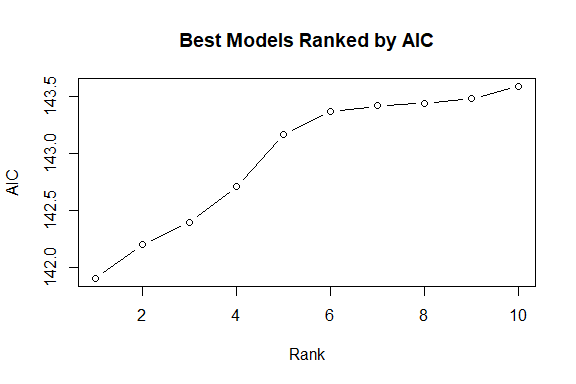plot(formulasumm, ptype = "variables")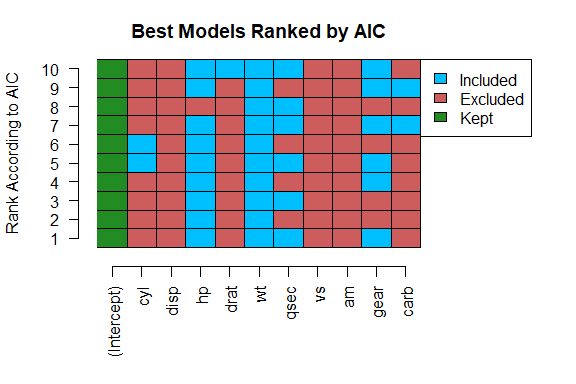## Getting best model
fit(formulasumm, which = 1)
#> Results from gamma regression with inverse link function
#> Using the formula mpg ~ hp + wt + qsec + gear
#>
#>               Estimate         SE      t  p.values
#> (Intercept) -4.691e-02  1.782e-02 -2.633   0.01384 *
#> hp          -6.284e-05  3.015e-05 -2.084   0.04675 *
#> wt          -9.485e-03  1.819e-03 -5.213 1.719e-05 ***
#> qsec         1.299e-03  7.471e-04  1.739   0.09348 .
#> gear         2.662e-03  1.652e-03  1.612   0.11870
#> ---
#> Signif. codes:  0 '***' 0.001 '**' 0.01 '*' 0.05 '.' 0.1 ' ' 1
#>
#> Dispersion parameter taken to be 0.0091
#> 32 observations used to fit model
#> (0 observations removed due to missingness)
#>
#> Residual Deviance: 0.29 on 27 degrees of freedom
#> AIC: 141.9
#> Algorithm converged in 3 iterations using Fisher's scoring

### 1.3.3 Using cutoff

• The cutoff argument can be used to find all models that have a metric value that is within cutoff of the minimum metric value found.
# Finding all models with a AIC within 2 of the best model
formulaVS <- VariableSelection(mpg ~ . ,data = cars, family = "gamma",
link = "inverse", type = "branch and bound",
showprogress = FALSE, metric = "AIC",
cutoff = 2)
formulaVS
#> Variable Selection Info:
#> ------------------------
#> Variables were selected using branch and bound selection with AIC
#> Found the top 16 models
#> The range of AIC values for the top 16 models is (141.9, 143.9)
#> Number of models fit: 110

## Getting summary and plotting results
formulasumm <- summary(formulaVS)
plot(formulasumm, type = "b")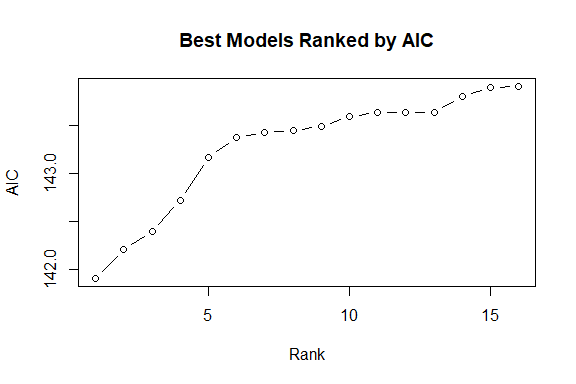plot(formulasumm, ptype = "variables")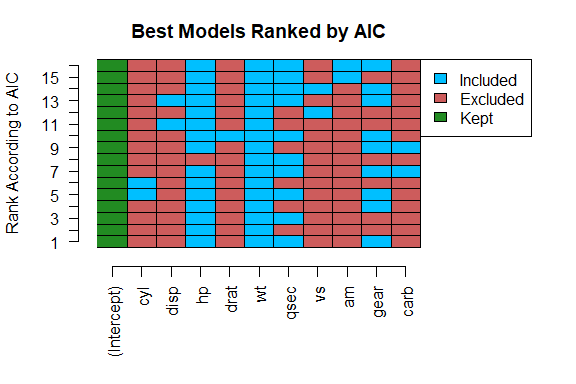## 1.4 Using keep

• Specifying variables via keep will ensure that those variables are kept through the selection process.
# Example of using keep
keepVS <- VariableSelection(mpg ~ . ,data = cars, family = "gamma",
link = "inverse", type = "branch and bound",
keep = c("hp", "cyl"), metric = "AIC",
showprogress = FALSE, bestmodels = 10)
keepVS
#> Variable Selection Info:
#> ------------------------
#> Variables were selected using branch and bound selection with AIC
#> Found the top 10 models
#> The range of AIC values for the top 10 models is (143.17, 145.24)
#> Number of models fit: 50

## Getting summary and plotting results
keepsumm <- summary(keepVS)
plot(keepsumm, type = "b")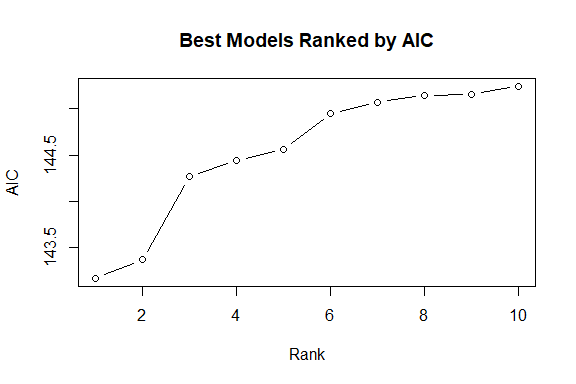plot(keepsumm, ptype = "variables")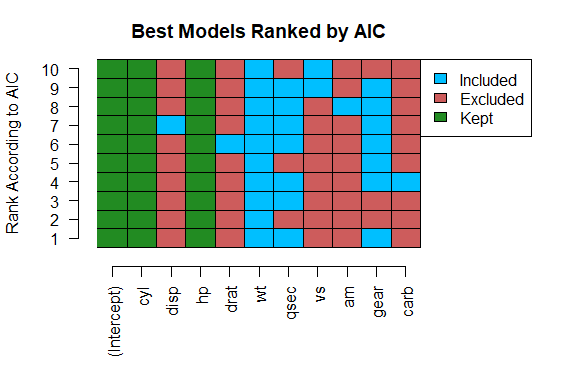## Getting final model
fit(keepsumm, which = 1)
#> Results from gamma regression with inverse link function
#> Using the formula mpg ~ cyl + hp + wt + qsec + gear
#>
#>               Estimate         SE       t  p.values
#> (Intercept) -6.464e-02  2.700e-02 -2.3940   0.02418 *
#> cyl          1.412e-03  1.642e-03  0.8603   0.39750
#> hp          -7.523e-05  3.321e-05 -2.2650   0.03205 *
#> wt          -1.037e-02  2.082e-03 -4.9830 3.517e-05 ***
#> qsec         1.816e-03  9.462e-04  1.9190   0.06603 .
#> gear         3.861e-03  2.155e-03  1.7910   0.08490 .
#> ---
#> Signif. codes:  0 '***' 0.001 '**' 0.01 '*' 0.05 '.' 0.1 ' ' 1
#>
#> Dispersion parameter taken to be 0.0089
#> 32 observations used to fit model
#> (0 observations removed due to missingness)
#>
#> Residual Deviance: 0.29 on 26 degrees of freedom
#> AIC: 143.17
#> Algorithm converged in 3 iterations using Fisher's scoring

## 1.5 Convergence issues

• It is not recommended to use branch and bound if the upper models do not converge since it can make the algorithm very slow.
• Sometimes when using backwards selection and all the upper models that are tested do not converge, no final model can be selected.
• For these reasons, if there are convergence issues it is recommended to use forward selection.